# Design of the Multistream Plate-Fin Heat Exchanger in the Air Separation Units

Design of the Multistream Plate-Fin Heat Exchanger in the Air Separation Units
Department of Mechanical Engineering, Faculty of Engineering, Shahid Chamran University of Ahvaz, Ahvaz 83151-61355, Iran

Corresponding Author Email:
banooni@scu.ac.ir
Page:
187-196
|
DOI:
https://doi.org/10.18280/ijht.410120
29 October 2022
|
Revised:
25 January 2023
|
Accepted:
10 February 2023
|
Available online:
28 February 2023
| Citation

OPEN ACCESS

Abstract:

The cold box with heat exchanger plate-fin (PFHE) has been applied in various applications, including air separation units (ASU). Therefore, this cryogenic industry has undergone a lot of development in recent years. Cryogenic technologies are utilized in many industrial procedures where they aid in heat recovery and reduce energy consumption. The multi-stream plate-fin heat exchanger (MSPFHE) is a substantial part of the air separation plant design. In this study, the energy contained in streams resulting from the distillation tower is used to cool the air entering this process to below freezing temperatures (-74 degrees Celsius). In the beginning, to find a suitable model and design, analytical solutions were used to find the heat duty and the optimal heat transfer area, including thermodynamic properties calculations, fin geometry dimensions, heat transfer calculations, and pressure drop calculations. The heat exchanger's design has also been evaluated analytically by calculating the fin efficiency and overall efficiency. A MATLAB code was written to achieve speed, accuracy, and consideration of all possible values. Input data from one of the studies in the literature was used as input for a case study. Finally, the rating of the design of the heat exchanger was done by one of the well-known software programs (EDR), and the results of this work were compared to those of previously published studies and they were found to be good and compatible.

Keywords:

thermodynamic properties, multistream plate-fin heat exchanger (MSPFHE), analytical solutions, aspen EDR, MATLAB

1. Introduction

Due to its small design, high level of efficiency (greater than 90%), and capacity to handle numerous gas streams, plate fin heat exchangers (PFHE) are used in the industry, particularly for cryogenic processes . One of the main applications is air separation units (ASU), which typically operate under the following conditions: a temperature difference between the cold and warm ends of between 100K and 300K; a pressure drop of between a few tens and hundreds of Pascal; and a temperature difference between the cold and warm streams of between a few Kelvin degrees. A variety of process and mechanical design elements have been examined, and innovative banking arrangements, exchanger surfaces, and flow compensation procedures have been offered in order to increase efficiency in a number of sources that deal with developing plate-fin heat exchangers . In a society that values the environment and saving energy, MSPFHEs are becoming more and more popular. On the other hand, plate-fin heat exchangers can be created and constructed for a wide range of applications and flow configurations. The MSPFHE countercurrent design, which has been utilized to handle cryogenic gases in air separation plants, is the primary subject of this work .

A classification system based on construction, operation, flow, and heat transfer was devised due to the enormous variety of heat exchanger combinations. The demand for improved performance parameters while maintaining the heat exchanger volume and weight relatively low gave rise to a new generation of heat exchangers known as compressed heat exchangers. Compact heat exchangers (CHE) (Extended Surface Exchangers of Heat) are one of the most important features of many cryogenic parts because they have a high heat transfer surface to volume ratio . Compact heat exchangers (CHE) are heat exchangers that have more than 700 m2/m3 of heat transfer surface area per unit volume (CHE). Compact heat exchangers are frequently used in gas-to-liquid (liquid-to-gas) and gas-to-gas heat exchange, where the large surface area also aids in boosting the low heat transfer coefficient associated with the gas stream. To lower the amount of energy used in these operations, a variety of effective and small pieces of equipment have been developed, with multi-stream plate-fin heat exchangers being one of the best options. As a result, this paper will address the design of this kind of exchanger.

Conventional heat exchangers, such shell and tube heat exchangers, are defined as heat exchangers with a prime surface that normally has an efficacy of 60% or less and a surface area density of the heat transfer that is typically 700 m2/m3 . Because the mass and volume of the box are constrained in particular applications and the increased effectiveness of the exchanger (up to about 98%) is crucial, a much smaller surface area has been assigned. Additionally, the coefficient of heat transmission in heat exchangers with gases or other fluids is quite low on one or both sides of the fluid, necessitating a high heat transfer surface area. The addition of extended surface (i.e., fins) and usage of the fins with a fin density that is as high as feasible on one fluid side or both of them, depending on design requirements, is a fairly typical way for enhancing exchanger compactness and surface area . An "extended surface exchanger" has been the name given to the resulting exchanger. By using thin-gauge material and properly sizing the core, the flow area is increased. In comparison to the unfinned surfaces, the extended surfaces' coefficient of heat transmission may be lower or higher. For example, internal tube fins enhance the surface area of the tube side, but interrupted (louvre, strip, and so on) fins show an increase in area as well as an increase in coefficient of heat transfer. Nevertheless, depending on the fin spacing, they can result in a modest reduction in the coefficient of heat transmission. In general, a decrease in the fins' coefficient of heat transmission follows an increase in fin density. The coefficient of heat transfer may rise by 2-4 times that of the comparable plain surface of the fin due to flow interruptions (such as those caused by louvred fins, offset strip fins, and so on) .

A compact heat exchanger (CHE), of which PFHE is one form, must have a surface-to-volume ratio greater than 700 m2/m3. Several plate-fin heat exchangers are 2-3 times more compact than traditional heat exchangers. They are able to transfer heat at such a rapid rate thanks to their special design. Figure 1 depicts a plate-fin heat exchanger with parallel plates (also known as separating sheets) joined by fins with different shapes. These fin tubes provide the flow channel for different fluid streams. We utilize thicker sidebars to close the flow path. Fins serve a special purpose in these heat exchangers. They support structural stiffness in addition to assisting with heat transmission.

## 1.png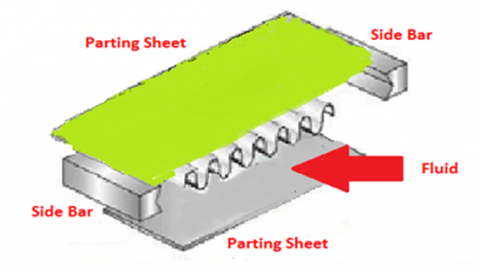Figure 1. A stack of fins is stacked between the separating sheets 

A plate-fin heat exchanger's fins aid in heat transfer in a number of different ways. The heat is initially transferred from a particular plate to the fluid stream when they are used as secondary surfaces (primary surface). When two neighbouring plates are joined by fins, a parallel path of heat transmission by conduction develops. Last but not least, common fin features may disturb the boundary layer and raise the local convective coefficient of heat transmission. The most typical fin sheets used in plate-fin heat exchangers are depicted in Figure 2 . The influence of operating parameters on plate-fin heat exchangers (PFHE) was examined by Patil and Rathod . The offset strip fins (OSF) with rectangular cross sections of the plate-fin heat exchanger are examined. MATLAB is used to create a steady-state model for the core dimensions of PFHE with a cross-flow configuration.

Tan et al.  studied the effects of properties on heat exchanger sizing design led to the identification of the crucial characteristics that had to be given top priority when creating a property model. Besides, the authors reported that even though viscosity has less significant impact compared to other properties, the larger deviation range of current viscosity models may lead to higher uncertainties in volume design and annual capital cost of heat exchanger. A technique was created by García-Castillo & Picón-Núñez  for figuring out the surface geometry that will produce the necessary pressure drop and heat transfer coefficient to meet the design criteria. The application of this methodology to a case study showed that a shell and tube heat exchanger of 227.4 m2, with the appropriate fin density using offset strip-fins, could be replaced by a plate and fin exchanger with any combination of height, width, and length in the ranges of 0–0.58 m, 0–0.58 m, and 0–3.59 m. This heat exchanger can be two-stream or multi-stream. Figure 3 shows the main structure of a multi-stream fin plate heat exchanger (MSPFHE). It has a stub pipe, a header tank, a distributed fin, a heat transfer fin, a partition plate, a sidebar, and a cover plate .

## 2.pngFigure 2. Various different forms of fins

## 3.png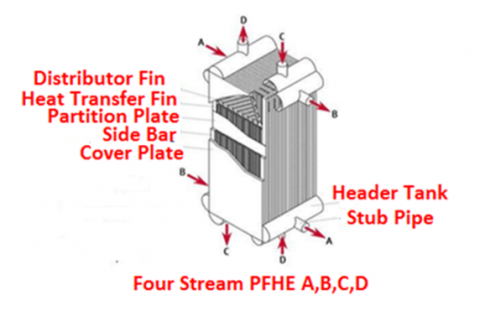Figure 3. Four-stream plate-fin heat exchanger 

The air is drawn into air separation units (ASU), which are found near factories. Filtered and cryogenically distilled air is used. Heat exchangers that operate at cryogenic temperatures are the most important components of an air separation plant. Heat exchangers with an efficiency of <90% could result in the failure of the plant, leading to the use of plate-fin heat exchangers with a large value of surface area per unit volume and low fluid pressure drop .

Although previous studies have discussed the design of the heat exchanger, there is a need for many more studies in an effort to obtain the optimal design. Therefore, the present study focuses on the plate-fin heat exchanger (PFHE) as a critical component in the design of air separation units. The energy contained in streams emanating from the distillation tower in the air separation unit (ASU) has been utilized to cool the air entering this operation in order to achieve reduced energy consumption. A multistream plate-fin heat exchanger (MSPFHE) has been designed based on one of the models. Firstly, MATLAB code using the technique of a certain model has been prepared, and the code obtained in some practical cases has been applied. This code has been used for sizing plate-fin heat exchangers in a number of cases with satisfactory results, which included thermodynamic property calculations, heat transfer calculations, pressure drop calculations, fin-type selection, and final heat exchanger dimensions. Here also, inconstant properties have been adopted as a fundamental element of obtaining the high effectiveness that this type requires. The study goal is to model and design a multistream plate-fin heat exchanger (MSPFHE) that can be applied practically without restrictions in the air separation unit cold box. Finally, the results have been validated and rated using the Aspen EDR software, based on the program's capacity to replicate the same environmental conditions that nature creates. An analytical solution and code were used to determine the heat exchanger's primary dimensions as well as the rest of the work's specifics. The efficiency was computed using the current findings and was discovered to be higher than 98%, which is required for this kind of application, as well as a confirmation of the findings by comparing them to prior literature. The current paper's outline is included, with sections 2—a mathematical model; 3—a design methodology; 4—design calculations and estimations; 5—results and discussion; and 6—conclusion.

2. Mathematical Model

2.1 Assumptions

All of the fluids in the heat exchanger, which have been depicted in Figure 4, are superheated, i.e., no change in the phase can occur. Also, the working hypotheses below make it less likely that heat will be transferred:

(1) The cold side (Nb) fin layer number has been assumed to be one higher than the hot side (Na).

(3) The study assume that the heat transfer coefficient, thermal capacities, and distribution area are all the same and stay the same.

(4) The thermal walls' resistance is ignored.

(5) Fouling is not noticeable for a heat exchanger of gas-to-gas; therefore, it is ignored.

(6) Axial heat conduction is negligible.

## 4.png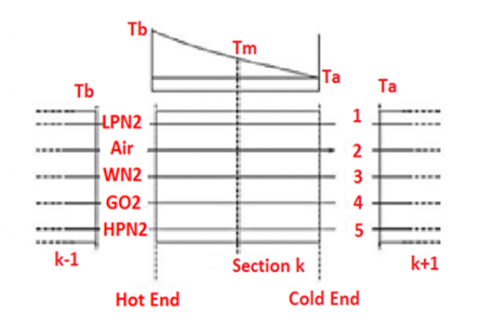Figure 4. Schematics of a section of the heat exchanger unit 

2.2 Thermal modeling

Figure 5 shows a diagram of a typical parallel and counterflow plate-fin heat exchanger with an offset strip fin, and Table 1 shows how to do thermal modelling of a plate-fin heat exchanger.

## 5.pngFigure 5. Schematic diagram of parallel and counter flow plate fin heat exchanger with rectangular offset strip fin 

Table 1. Formulation related to thermal modeling 

 NO Equations Remarks 1 $\varepsilon=1-\exp \left\{\left(\frac{1}{C^*}\right) N T U^{0.22} *\left(\exp \left\{-C^*(N T U)^{0.78}-1\right)\right\}\right.$ Effectiveness of counter flow HE 2 $N T U_{\max }=\frac{U A_{\text {tot }}}{C_{\min }}$ Number of transfer units 3 $C^*=\frac{C_{\min }}{C_{\max }}$ Total heat capacity ratio 4 $U=\frac{1}{\frac{1}{\left(h \eta_s\right)}+\frac{1}{\frac{A_\,{t o t, h}}{A_\,{t o t, c}}\,\left(h \eta_s\right)\,_h}}$ Overall heat transfer coefficient 5 $\beta=\frac{A_{c e l l}}{V_{c e l l}}=\frac{2\left(b-t_f\right) x+2\left(c-t_f\right) x+x\left(b-t_f\right) t_f+c t_f}{b c x}$ Surface area density 6 $\dot{Q}=C_h\left(T_{h, 1}-T_{h, 2}\right)$ Rate of heat transfer in hot side 7 $\dot{Q}=C_c\left(T_{c, 2}-T_{c, 1}\right)$ Rate of heat transfer in cold side 8 $V_p=L_c L_h b N$ Volume between all two plates 9 $N=\frac{L_n-b_c+2 t_w}{b_h+b_c+2 t_w}$ Total passages 10 $\eta_s=1-\frac{A_f}{A_{\text {cell }}}\left(1-\eta_f\right)$ Overall surface efficiency

## 6.png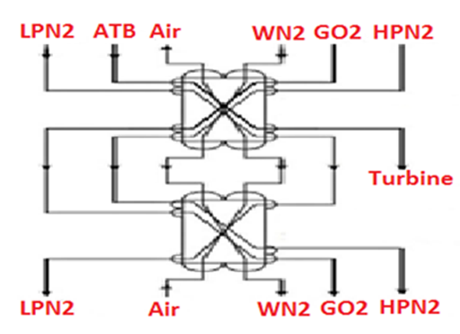Figure 6. Hot and cold streams in recuperative heat exchangers 

Heat exchange occurs between cold products and hot air, as shown in Figure 6. In the case study (data shown in Table 2), multistream heat exchangers have been used to cool the incoming warm air stream with the cold gases produced by distillation towers in the air separation unit, reducing the need for external refrigeration.

Table 2. Design data provided by Boehme et al. 

 Stream Air WN2 GO2 LPN2 HPN2 Mass Flow Rate(kg/h) 17,258.6 10,958.3 3432.8 1798.6 1001.1 Cold End Pressure (kPa) 678.3 121.6 135.2 128.4 544.5 Cold End Temp. (K) 195.7 193.15 193.15 193.15 193.15 Hot End Temp. (K) 311.15 308.15 308.15 308.15 308.15

2.3 Dimensioning of the heat exchanger

The dimensions of the HEX need to be decided so that the heat exchanger may operate soundly in its operating pressure ambit. Which is why the maximal limits of the width, height, and length should not be exceeded by 1.2 m, 1.2 m, and 6.2 m . The length has to be in the flow direction. The width value has been assumed within the limit that has been characterised before, and then other dimensions are estimated according to the width.

2.4 Fluid thermo physical properties

The important step in the design process of HEX represents a definition of the physical characteristics of the materials . Regarding solid materials, just the equations of conduction are solved for steady-state problems, so the value of thermal conductivity is wanted as an average quality. However, fluid characteristics like thermal conductivity, density, and viscosity are needed for design calculations. There are two assumptions, the first one depends on the independence of temperature and the other one depends on the dependence of temperature. In the present study, the thermophysical characteristics of the fluids have been assumed to be temperature-independent. By using the ASPEN HYSES simulation program, it can get the properties in Tables 3, 4, 5, and 6.

Table 3. cp of five streams calculated by ASPEN HYSES

 Stream cp (J/kg.C) In Out Average LPN2 1026.66 1044.16 1035.415 Air 1004.04 1003.69 1003.87 WN2 1026.31 1044.07 1035.19 GO2 883.35 916.66 900.01 HPN2 1048.53 1051.69 1050.11

Table 4. Density of five streams calculated by ASPEN HYSES

 Stream Density (kg/m3) In Out Average LPN2 2.2487 1.2779 1.7633 Air 7.5737 12.1993 9.8865 WN2 2.1292 1.2230 1.6761 GO2 2.7087 1.6237 2.1662 HPN2 9.6606 5.9265 7.7935

Table 5. Viscosity of five streams calculated by ASPEN HYSES

 Stream Viscosity (Pa.s) E-05 In Out Average LPN2 1.2792 1.8800 1.5800 Air 1.9550 1.3500 1.6500 WN2 1.2791 1.8800 1.5800 GO2 1.4290 2.1500 1.7900 HPN2 1.2903 1.8800 1.5800

Table 6. Thermal Conductivity of five streams calculated by ASPEN HYSES

 Stream Thermal Conductivity (W/m.k) In Out Average LPN2 1.79E-02 2.65E-02 2.22E-02 Air 2.59E-02 2.59E-02 2.59E-02 WN2 1.79E-02 0.026511 2.22E-02 GO2 1.76E-02 2.71E-02 2.24E-02 HPN2 1.83E-02 2.67E-02 2.25E-02

2.5 Analytical solution

## 1.png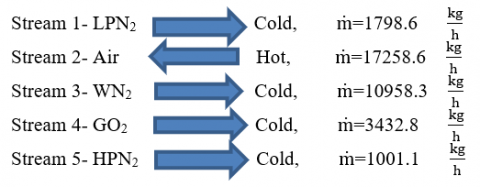3. Calculations and Estimations of Design

3.1 Estimation of heat duty

TAir in=311.15K, TAir out=195.7K

$C_{a i r}=\dot{m}_{A i r} C p_{A i r}=4812.608 \mathrm{~W} /{ }^{\circ} \mathrm{C}$

TAir=311.15-195.7=115.45 K=115.45℃

QAir=CAir ∆TAir=555.616 kW

Based on assume, the problem steady state: $Q_{A i r}=Q_{L P N_2}+Q_{W N_2}+Q_{G O_2}+Q_{H P N_2}$.

·Heat lost by Air=Heat gained by 4 gases

·Heat duty=555.616 Kw

·In the same way, it calculates the heat loads of other streams as shown in Table 7.

Table 7. Calculation the heat loads of other streams

 Stream Air WN2 GO2 LPN2 HPN2 Heat load (kW) 555.616 362.38 98.50 59.50 33.58

3.2 Estimation of overall coefficient heat transfer

By use diagram in Figure 7 below, U=85 W/m2℃.

## 7.pngFigure 7. Diagram to calculate the overall heat transfer coefficient 

3.3 Estimation of log mean temperature difference

## 8.png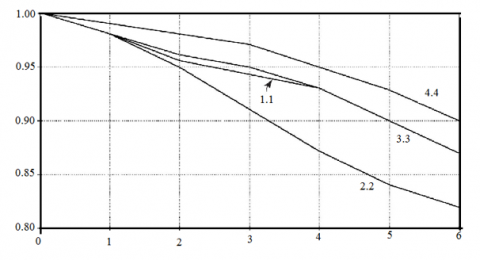Figure 8. Diagram to calculate the correction factor 

Based on Figure 8, the estimation of the Log Mean Temperature Difference is shown below:

·For hot stream (Air): Th in=311.15 K, and Th out=195.7 K

·For cold stream, (There are four cold streams):

$\begin{gathered}T_{\text {c in }}=T_{L P N_2}=T_{W N_2}=T_{G O_2}=T_{H P N_2}=193.15 \mathrm{~K} \\ T_{\text {cout }}=T_{L P N_2}=T_{W N_2}=T_{G O_2}=T_{H P N_2}=308.15 \mathrm{~K} \\ \Delta T_{\text {lm }}=\frac{\left(T_{\text {hin }}-T_{\text {cout }}\right)-\left(T_{\text {hout }}-T_{\text {cin }}\right)}{\ln \left[\frac{\left(T_{h \text { hin }}-T_{\text {cout }}\right)}{\left(T_{\text {hout }}-T_{\text {cin }}\right)}\right]}=2.769{ }^{\circ} \mathrm{C} \\ N T U=1.06\end{gathered}$

·By use diagram in Figure 8 below, Ft=0.97.

·Estimate (∆Tm), ∆Tm=∆Tlm*Ft=2.67℃.

3.4 Estimation of area of heat transfer

The optimum required area for heat transfer:

$A_{\text {air }}=\frac{Q}{U * \Delta T_m}=2447.648 \mathrm{~m}^2$

Then, In the same way, we calculate the area of heat transfer of other streams as shown in Table 8:

Table 8. Calculation of the area of heat transfer of other streams

 Stream Air WN2 GO2 LPN2 HPN2 Area (m2) 2447.648 1596.3 433.9 262.11 147.9

3.5 Estimations of geometry of the heat exchanger

The size of a brazed aluminum plate-fin heat exchanger shall be specified by width W, stacking height H, and length L of the rectangular block. By trial and error method, the authors calculated the suitable length (L2), L2=4.03 m and height (L3): L3=1.146 m. Figure 9 display fin geometries of the case study which was used in the present work.

## 9.png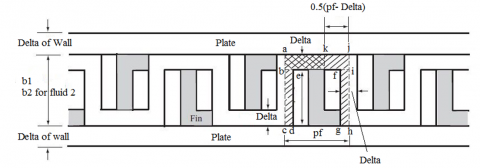Figure 9. Offset strip fin geometry under consideration 

The allowed ranges of the design variables are as follows:

1. Aluminum heat exchanger can be made up to width (L1), 1.2 m×height (L3), 1.2 m cross section, and length (L2), 6.2 m (As the first attempt) .
2. Fin height, b (mm): 2≤b≤ 12. 
3. Fin thickness, δ (mm): 0.15≤δ≤ 0.7. 
4. Plate thickness (δw) (mm): 0.5≤δ≤0.3.
5. Frequency of fin (n): 100 ≤n≤1000. 
6. Offset length, x (mm): 1 ≤x≤ 10. 
7. Fin layers number (Np): 1≤Np≤200. 

Accordingly, it can determine the design information of the geometry of the heat exchanger that is shown below in Table 9 as follows:

Table 9. Design of geometry details

 Particulars Hot side Cold side Thickness of fins, (δ) (mm) 0.25 0.25 Thickness of plate (δw) (mm) 2 2 Height of fin (b) (mm) 6 5 Length of fin, (λ) (mm) 8 7 Density of fin (Nf) 402 672 Width of fin, (pf) (mm) 1.8 1.488

3.5.1 Calculations of hot side

·The number of passages for the hot fluid side be: $\mathrm{N}_{\mathrm{p}}=\frac{\mathrm{L}_3-\mathrm{b}_2-2 \delta_{\mathrm{W}}}{\mathrm{b}_1+\mathrm{b}_2+2 \delta_{\mathrm{W}}}=63$

·The total number of fins for hot fluid are calculated by: $\mathrm{n}_{\mathrm{f} 1}=\frac{\mathrm{L}_1}{\mathrm{p}_{\mathrm{f}}} * \mathrm{~N}_{\mathrm{p}}=42 * 10^3$

·The total primary area (Ap) for hot fluid (air) calculated by:

\begin{aligned} \mathrm{A}_{\mathrm{p}, \text { hot }}= & {\left[2 \mathrm{~L}_1 \mathrm{~L}_2 \mathrm{~N}_{\mathrm{p}}-2 \delta \mathrm{L}_2 \mathrm{n}_{\mathrm{f} 1}\right]+\left[2 \mathrm{~b}_1 \mathrm{~L}_2 \mathrm{~N}_{\mathrm{p}}\right]+\left[2\left(\mathrm{~b}_2+\right.\right.} \\ & \left.\left.2 \delta_{\mathrm{w}}\right) \mathrm{L}_1\left(\mathrm{~N}_{\mathrm{p}}+1\right)\right] \mathrm{A}_{\mathrm{p}, \text { hot }}=529.135 \mathrm{~m}^2\end{aligned}

·Calculate the number of offset strip fins for hot fluid (per the number of fins) by: $\mathrm{n}_{\mathrm{off}, \text { hot }}=\frac{L_2}{\lambda_1}=500$

·The total fin area Af1 for hot fluid is obtained using Equation:

$\begin{gathered}\mathrm{A}_{\mathrm{f}, \text { hot }}=2\left(\mathrm{~b}_1-\delta\right) \mathrm{L}_2 \mathrm{n}_{\mathrm{f} 1}+2\left(\mathrm{~b}_1-\delta\right) \delta \mathrm{n}_{\mathrm{off} 1} \mathrm{n}_{\mathrm{f} 1}+ \\ \left(\mathrm{p}_{\mathrm{f} 1}-\delta\right) \delta\left(\mathrm{n}_{\mathrm{off} 1}-1\right) \mathrm{n}_{\mathrm{f} 1}+2 \mathrm{p}_{\mathrm{f} 1} \delta \mathrm{n}_{\mathrm{f} 1} \\ \mathrm{~A}_{\mathrm{f}, \text { hot }}=2015.02 \mathrm{~m}^2 \\ \mathrm{~A}_{\text {total,hot side }}=\mathrm{A}_{\mathrm{p}, \text { hot }}+\mathrm{A}_{\mathrm{f}, \text { hot }}=2544.159 \mathrm{~m}^2\end{gathered}$

This is larger required by: $\frac{2544.159-2447.648}{2447.648}=3.94 \%$ (This percentage is acceptable to compensate any loss of efficiency of heat transfer). Other geometrical characteristics of interest are:

The free-flow area on the air side is calculated by subtracting the frontal area on the air side from the area blocked by the fins at the core's entry on that side.

$\mathrm{A}_{\mathrm{c}, \text { hot }}=\left(\mathrm{b}_1-\delta\right) *\left(\mathrm{p}_{\mathrm{f}}-\delta\right) * \mathrm{n}_{\mathrm{f}}=0.37 \mathrm{~m}^2$

·The frontal area for hot stream: $\mathrm{A}_{\mathrm{fr}}=\mathrm{L}_1 * \mathrm{~L}_3=1.375 \mathrm{~m}^2$

·The porosity $(\sigma)$: $\sigma=\frac{\mathrm{A}_{\mathrm{c}}}{\mathrm{A}_{\mathrm{fr}}}=0.27$

·The surface area density (β) is: $\beta=\frac{A_{\text {hot total }}}{\mathrm{b}_1 \mathrm{~N}_{\mathrm{b}} \mathrm{L}_1 \mathrm{~L}_2}=1392 \frac{\mathrm{m}^2}{\mathrm{~m}^3}$

·The hydraulic diameter (Dh) is: $\mathrm{D}_{\mathrm{h}, \text { hot side }}=\frac{4 * \mathrm{~L}_2 * \mathrm{~A}_{\mathrm{c}}}{\mathrm{A}_{\text {hot total }}}=0.0023 \mathrm{~m}$

3.5.2 Calculations of cold side

·The number of passages for the cold side=Np+1=64

·The total number of fins for cold side are calculated by: $\mathrm{n}_{\mathrm{f} 2}=\frac{\mathrm{L}_1}{\mathrm{p}_{\mathrm{f}}}\left(\mathrm{N}_{\mathrm{p}}+1\right)=51.599 * 10^3$

·The total primary area (Ap)for cold side calculated by: $\mathrm{A}_{\mathrm{p}, \text { cold }}=\left[2 \mathrm{~L}_1 \mathrm{~L}_2\left(\mathrm{~N}_{\mathrm{p}}+1\right)-2 \delta \mathrm{L}_2 \mathrm{n}_{\mathrm{f} 2}\right]+\left[2 \mathrm { b } _ { 2 } \mathrm { L } _ { 2 } \left(\mathrm{N}_{\mathrm{p}}+\right.\right.1) ]+\left[2\left(\mathrm{~b}_1+2 \delta_{\mathrm{w}}\right) \mathrm{L}_1 \mathrm{~N}_{\mathrm{p}}\right]$

$A_{\mathrm{p}, \mathrm{cold}}=519.12 \mathrm{~m}^2$

·Calculate the number of offset strip fins: $\mathrm{n}_{\mathrm{off}, \mathrm{cold}}=\frac{\mathrm{L}_2}{\lambda_2}=575$

·The total fin area Af1 for hot fluid is obtained using Equation:

$\begin{gathered}\mathrm{A}_{\mathrm{f}, \text { cold }}=2\left(\mathrm{~b}_2-\delta\right) \mathrm{L}_2 \mathrm{n}_{\mathrm{f} 2}+2\left(\mathrm{~b}_2-\delta\right) \delta \mathrm{n}_{\mathrm{off} 2} \mathrm{n}_{\mathrm{f} 2}+ \\ \left(\mathrm{p}_{\mathrm{f} 2}-\delta\right) \delta\left(\mathrm{n}_{\mathrm{off} 2}-1\right) \mathrm{n}_{\mathrm{f} 2}+2 \mathrm{p}_{\mathrm{f} 2} \delta \mathrm{n}_{\mathrm{f} 2} \\ \mathrm{~A}_{\mathrm{f}, \mathrm{cold}}=2053.58 \mathrm{~m}^2 \\ \mathrm{~A}_{\mathrm{t}}=\mathrm{A}_{\mathrm{p}, \mathrm{cold}}+\mathrm{A}_{\mathrm{f}, \mathrm{cold}} \\ \mathrm{A}_{\text {total,cold side }}=519.12+2053.58=2574.26 \mathrm{~m}^2\end{gathered}$

This is larger required by: $\frac{2574.26-2447.648}{2447.648}=5 \%$, and other geometrical characteristics of interest are:

·The free-flow area on the cold side: $\mathrm{A}_{\mathrm{c}, \mathrm{cold}}=\left(\mathrm{b}_2-\delta\right) *\left(\mathrm{p}_{\mathrm{f} 2}-\delta\right) * \mathrm{n}_{\mathrm{f} 2}=0.3 \mathrm{~m}^2$

·The frontal area for cold streams: Afr=L1*L3=1.375 m2

·The porosity for cold streams: $\sigma=\frac{A_c}{A_{\mathrm{fr}}}=0.218$

·The surface area density for cold streams:

$\beta=\frac{\mathrm{A}_{\text {cold total }}}{\mathrm{b}_2 \mathrm{~N}_{\mathrm{b}} \mathrm{L}_1 \mathrm{~L}_2}=\frac{2574.26}{5 * 10^{-3} * 63 * 1.2 * 4.03}=1689 \frac{\mathrm{m}^2}{\mathrm{~m}^3}$

The hydraulic diameter (Dh), $\mathrm{D}_{\mathrm{h}, \text { cold side }}=\frac{4 * \mathrm{~L}_2 * \mathrm{~A}_{\mathrm{c}}}{\mathrm{A}_{\text {cold total }}}=0.00188 \mathrm{~m}$.

3.5.3 Calculations of pressure drops

It can estimate pressure drop for hot side (air stream):

·Calculate the volumetric flow rate of air, $\dot{\mathrm{V}}_{\mathrm{air}}=\frac{\dot{\mathrm{m}}_{\mathrm{air}}}{\rho_{\mathrm{air}}}=0.485 \mathrm{~m}^3 / \mathrm{s}$

·Calculate the velocity of air, $\mathrm{u}_{\mathrm{air}}=\frac{\mathrm{V}_{\mathrm{air}}}{\mathrm{A}_{\mathrm{c} 1}}=1.31 \frac{\mathrm{m}}{\mathrm{s}}$

·Calculate the Reynolds number, $\operatorname{Re}=\frac{\rho \mathrm{uD}_{\mathrm{h}}}{\mu}=1805.97$

·Calculate the friction factor $\mathrm{f}_{\text {hot }}($ For $120 \leqslant \operatorname{Re} \leqslant 104)$ on the hot side (air) is:

$\mathrm{f}_1=9.6243 \mathrm{Re}^{-0.7422}\left(\frac{\mathrm{p}_{\mathrm{f}}-\delta}{\mathrm{b}-\delta}\right)^{-0.1856}\left(\frac{\delta}{\lambda}\right)^{0.3053}\left(\frac{\delta}{\mathrm{p}_{\mathrm{f}}-\delta}\right)^{-0.2659}$$\begin{gathered}{\left[1+7.669 * 10^{-8} \operatorname{Re}^{4.429}\left(\frac{\mathrm{p}_{\mathrm{f}}-\delta}{\mathrm{b}-\delta}\right)^{0.92}\left(\frac{\delta}{\lambda}\right)^{3.767}\left(\frac{\delta}{\mathrm{p}_{\mathrm{f}}-\delta}\right)^{0.236}\right]^{0.1}} \\ {\left[\text { Get: }\left(\frac{\mathrm{p}_{\mathrm{f}}-\delta}{\mathrm{b}-\delta}\right)=0.27,\left(\frac{\delta}{\lambda}\right)=0.031,\left(\frac{\delta}{\mathrm{p}_{\mathrm{f}}-\delta}\right)=0.16\right]} \\ \therefore \mathrm{f}_1=0.033\end{gathered}$

·Calculate the mass velocity $\left(G_{\text {air }}\right)$ on the hot side, $\mathrm{G}_{\text {air }}=\frac{\dot{\mathrm{m}}_{\mathrm{air}}}{\mathrm{A}_{\mathrm{c}}}=12.95 \frac{\mathrm{kg}}{\mathrm{m}^2 \mathrm{~s}}, \rho_{\text {in }}=7.573762\left(\mathrm{~kg} / \mathrm{m}^3\right), \rho_{\text {out }}=9.886560384\left(\mathrm{~kg} / \mathrm{m}^3\right)$

·Calculate the ($\left(\rho_{m}\right),\left(\frac{1}{\rho_{\mathrm{m}}}\right)=\frac{1}{2} *\left(\frac{1}{\rho_{\text {in }}}+\frac{1}{\rho_{\text {out }}}\right), \rho_{\mathrm{m}}=8.576\left(\mathrm{~kg} / \mathrm{m}^3\right)$

\begin{aligned} & \mathrm{K}_{\mathrm{in}}=0.40461538+0.00817016 \sigma-0.41317016 \sigma^2 =0.376 \\ & \mathrm{~K}_{\mathrm{out}}=0.99895105-1.96088578 \sigma+0.96270396 \sigma^2 =0.539 \\ & \Delta \mathrm{P}=\frac{\mathrm{G}^2}{2 * \rho_{\text {in }}} *\left[\left(1-\sigma^2+\mathrm{K}_{\mathrm{in}}\right)+2\left(\frac{\rho_{\text {in }}}{\rho_{\text {out }}}-1\right)+\frac{4 \mathrm{fL}_2}{\mathrm{D}_{\mathrm{h}}}\right. \\ & \left.*\left(\frac{\rho_{\mathrm{in}}}{\rho_{\mathrm{m}}}\right)-\left(1-\sigma^2-\mathrm{K}_{\text {out }}\right) *\left(\frac{\rho_{\text {in }}}{\rho_{\text {out }}}\right)\right] \\ & \therefore \Delta \mathrm{P}_{\mathrm{air}}=2267 \mathrm{~Pa} \\ & \end{aligned}

To estimate pressure drop for cold side, with same way calculated the pressure drop for cold side streams as shown in Table 10.

Table 10. Pressure drop for cold side streams

 Streams Velocity (m⁄s) Re ∆P (Pa) LPN2 0.943 197.85 1487 WN2 6.06 1208.6 11145 GO2 1.46 332.2 1944.96 HPN2 0.118 108.73 101
4. Results and Discussion

4.1 Rating of design (The efficiency)

Based on the above calculations, it can calculate the efficiency of the system under consideration:

Using data of air stream, where Cpair=1003.87, Prair=0.64, and when 400≤Re≤5500, \begin{aligned} & \mathrm{j}=2.11497 * 10^{-2}-1.02089 * 10^{-5} \mathrm{Re}+2.37311 * 10^{-9} \mathrm{Re}^2-1.89734 * 10^{-13} \mathrm{Re}^3, \mathrm{j}=0.00933\end{aligned}.

·Calculate the heat transfer coefficient $(\alpha): \alpha=\mathrm{jGc}_{\mathrm{p}} \operatorname{Pr}^{-2 / 3}=163.32 \frac{\mathrm{w}}{\mathrm{m}^2 \cdot \mathrm{k}}$

where, kf is thermal conductivity, $\mathrm{k}_{\mathrm{f}}=88: 251 \frac{\mathrm{w}}{\mathrm{m} \cdot \mathrm{K}}$ , for all alloys .  kAverage)=169.5$\frac{w}{m \cdot K}$

·Calculate fin parameter (m), $\mathrm{m}=\sqrt{\frac{2 \alpha}{\mathrm{k}_{\mathrm{f}} \delta}\left(1+\frac{\delta}{\lambda}\right)}=89.158$

·Calculate the fin profile length, $\mathrm{L}_{\mathrm{f}}=\frac{\mathrm{b}_1}{2}-\delta=0.00275 \mathrm{~m}$

·Calculate the fin efficiency (ηf), $\eta_{\mathrm{f}}=\frac{\tanh \left(\mathrm{mL}_{\mathrm{f}}\right)}{\mathrm{mL}_{\mathrm{f}}}=98 \%$

The overall surface efficiency $\left(\eta_{\text {overal HT }}\right)=1-\frac{\mathrm{A}_{\mathrm{f}}}{\mathrm{A}_{\mathrm{HT}}}\left(1-\eta_{\mathrm{f}}\right)=98.4 \%$.

4.2 MATLAB code

A computer code has been written based on the steps summed up in the preceding sections. The program has been coded using the MATLAB language and has a mainline and seven sub-routines. A flowchart of code work (Figure 10) shows a plate-fin multi-stream heat exchanger design. The program calculates the optimum required area of heat transfer, fin geometry, and overall exchanger sizing. The advantage of using code lies in its capability for handling every one of the streams in the exchanger in an individual manner and providing the profiles of the pressure, temperature, heat transfer coefficients, Reynolds number, and so on, at the stage of the sizing. The code included sizing and rating processes.

## 10.pngFigure 10. Flow chart of the code work

4.3 Validation the results by aspen EDR software

The validation of the data gathered in the case study to design a heat exchanger is presented in this part. The outcomes of the chosen design technique are evaluated against those obtained using advanced simulation tools. The rigorous simulation software for comparison was Aspen Exchanger Design and Rating (Aspen EDR) Version 10.0. EDR provides detailed simulations of multi-stream plate-fin heat exchangers based on thermal-hydraulic correlations.

The findings obtained using data from Boehme et al.  have been validated and compared with those obtained using the rigorous simulation tool EDR. In this case study, the number of streams is five. One stream is hot and the others are cold. At the start of the EDR menu, you can choose the computation mode as well as the number of streams and project title, as shown in Figure 11.

## 11.png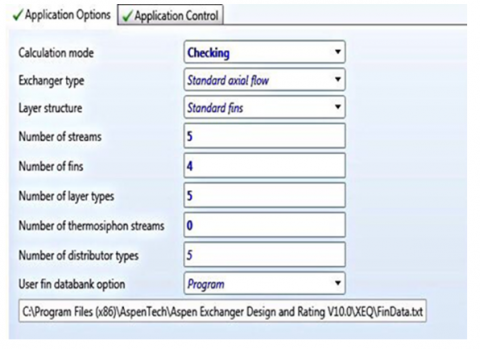Figure 11. The selecting of the calculation mode and the details of number of streams in EDR software

The data entry process for the streams in case one is shown in Figure 12.

## 12.png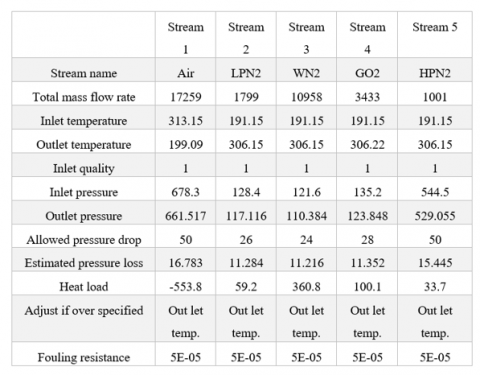Figure 12. The data entry process of mass flow rate, temperatures, and inlet pressures of the streams in EDR software

After assembling the input data, it is executed with the display of errors and warnings (if any), and the results may be seen in a summary or in detail. Figures 13 and 14 are shown as follows.

## 13.pngFigure 13. The full results-1 in detail

## 14.pngFigure 14. The full results-2 in detail

4.4 Comparison with previous results

In this section, comparison of data from the current study and a previous study for a heat exchanger by Boehme et al. . Table 11 displays the comparison results.

Table 11. Comparison of data from the current study and a previous study for a heat exchanger by Boehme et al. 

 Stream Air WN2 GO2 LPN2 Hot end temperature (K) 313.05 313 307.76 306 307.19 306 307.14 306.07 Previous study Present design Cold end temperature (K) 198.48 198.98 193.15 191 193.15 191 193.15 191 Previous study Present design Heat transfer area (m2) 1453.98 2447.64 1453.9 1596.3 485.34 433.9 259.32 262.11 Previous study Present design Rate of heat transfer (kW) 555.21 555.616 363.97 362.38 98.67 98.50 59.43 59.50 Previous study Present design Core pressure drop (Pa) 3861 2264 10333 6819 5581 2183 10220 10680 Previous study Present design
5. Conclusions

Although the design of the heat exchanger has been covered in earlier research, there is still a need for many more studies to find the best design. This is the motivation for the current study. The plate-fin heat exchanger (PFHE), which is a crucial part of the design of air separation units, is the subject of the current study. In order to reduce energy consumption, the air entering this operation has been cooled using energy from streams coming from the distillation tower in the air separation unit (ASU). Based on one of the models, a multistream plate-fin heat exchanger (MSPFHE) was created. In the beginning, MATLAB code was created utilizing a certain model's technique, and it was then applied to several real-world scenarios. This code has been successfully used to size plate-fin heat exchangers in a range of situations, including calculations of thermodynamic property, heat transfer, pressure drop, fin-type selection, and final heat exchanger dimensions. In this case as well, inconsistency has been chosen as a key component of achieving the high efficacy that this kind demands. The purpose of the project is to develop and create a multistream plate-fin heat exchanger (MSPFHE) that may be used in the cold box of an air separation unit practically and without limitations. Finally, the effectiveness of the Aspen EDR software in simulating the same environmental circumstances that nature produces has been evaluated and the results have been validated. The main dimensions of the heat exchanger and details of the rest of the work were determined using an analytical solution and code.

The heat exchanger was designed, and the main dimensions of the exchanger, as well as the rest of the work details, were obtained using an analytical solution and code. Based on the current findings, the efficiency was calculated and found to be higher than 98%, which is required in this type of application, as well as a validation of the results by comparing them with previous literature. On the other hand, an investigation using the EDR program was conducted. Finally, the results of this work were compared to those of previously published studies, and the validity of the proposed program showed that the results were very acceptable.

Nomenclature
 A Area (m2) Af Total fin area (m2) Ap Total primary area (m2) At Total area (m2) b Fin height (m) cp Specific heat ( $\left(\mathrm{Jkg}^{-1} \mathrm{~K}^{-1}\right)$ ) C Heat capacity ($\mathrm{WK}^{-1}$) Dh Hydraulic diameter (m) f Fanning friction factor j Colburn factor Re Reynolds number G Mass flow velocity ($\mathrm{kgs}^{-1} \mathrm{~m}^{-2}$) Lf Fin profile length (m) L1 Length of the core HE (m) δw plate thickness (m) μ Dynamic viscosity coefficient ( $\mathrm{Nsm}^{-2}$) ρ Density ($\mathrm{kgm}^{-3}$) ηf Fin efficiency L2 Width of the core of HE (m) L3 Core height(m) m Fin parameter ($m^{-1}$) $\dot{m}$ Mass flow rate ($\mathrm{kgs}^{-1}$) nf Number of fins noff Number of offset fins ∆P Pressure drop (Pa) Np Number of passages Nf Fin densities (Fins/m) P Pressure (Pa) Pr Prandtl number U Overall heat transfer Coefficient ($\mathrm{Wm}^{-2} \mathrm{~K}^{-1}$) T Temperature (K) u Velocity $\left(\mathrm{m}\, \mathrm{s}^{-1}\right)$ pf Fin pitch (m) α Heat transfer coefficient $\left(\mathrm{Wm}^{-2} \mathrm{~K}^{-1}\right)$ δ Fin thickness (m) ηoveral HT Overall surface fin efficiency
References

 Mohammed Hussein, H.A., Zulkifli, R., Mahmood, W. M.F.B.W., Ajeel, R.K. (2022). Structure parameters and designs and their impact on performance of different heat exchangers: A review. Renewable and Sustainable Energy Reviews, 154: 111842. https://doi.org/10.1016/j.rser.2021.111842

 Ajeel, R.K., Sopian, K., Zulkifli, R. (2021). Thermal-hydraulic performance and design parameters in a curved-corrugated channel with L-shaped baffles and nanofluid. Journal of Energy Storage, 34: 101996. https://doi.org/10.1016/j.est.2020.101996

 Prasad, B.S.V., Gurukul, S.M.K.A. (1987). Differential method for sizing multistream plate fin heat exchangers. Cryogenics, 27(5): 257-262. https://doi.org/10.1016/0011-2275(87)90032-4

 Ajeel, R.K., Salim, W.I., Hasnan, K. (2019). Design characteristics of symmetrical semicircle-corrugated channel on heat transfer enhancement with nanofluid. International Journal of Mechanical Sciences, 151: 236-250. https://doi.org/10.1016/j.ijmecsci.2018.11.022

 Shah, R.K., Sekulic, D.P. (2003). Fundamentals of Heat Exchanger Design. John Wiley & Sons.

 Mohammed Hussein, H.A., Zulkifli, R., Mahmood, W.M.F.B.W., Ajeel, R.K. (2022). Effects of design parameters on flow fields and heat transfer characteristics in semicircle oblique-finned corrugated. International Communications in Heat and Mass Transfer, 135: 106143. https://doi.org/10.1016/j.icheatmasstransfer.2022.106143

 Sahoo, M. (2015). Experimental and numerical studies on plate fin heat exchanger. Doctoral dissertation.

 Das, P.K., Ghosh, I. (2012). Thermal design of multistream plate fin heat exchangers—A state-of-the-art review. Heat transfer engineering, 33(4-5): 284-300. https://doi.org/10.1080/01457632.2012.611451

 Patil, N., Rathod, M. (2012). Numerical investigation on effect of operating parameters on plate fin heat exchanger. Proc. World Congr. Eng, 3: 4-9.

 Tan, Y., Nookuea, W., Li, H., Thorin, E., Yan, J. (2019). Impacts of thermos-physical properties on plate-fin multi-stream heat exchanger design in cryogenic process for CO2 capture. Applied Thermal Engineering, 149: 1445-1453. https://doi.org/10.1016/j.applthermaleng.2018.12.066

 García-Castillo, J., Picón-Núñez, M. (2021). Physical dimensions as a design objective in heat transfer equipment: The case of plate and fin heat exchangers. Energies, 14(8): 2318. https://doi.org/10.3390/en14082318

 Guo, K., Zhang, N., Smith, R. (2015). Optimisation of fin selection and thermal design of counter-current plate-fin heat exchangers. Applied Thermal Engineering, 78: 491-499. https://doi.org/10.1016/j.applthermaleng.2014.11.07

 Cuzic, E.M., Viorel, P.O.P.A., Popa, C.L. (2013). Study of the main heat exchanger in a cryogenic air separation unit. Engineering, Physics, Corpus ID: 51744505

 Boehme, R., Parise, J.A.R., Marques, R.P. (2003). Simulation of multistream plate–fin heat exchangers of an air separation unit. Cryogenics, 43(6): 325-334. https://doi.org/10.1016/S0011-2275(03)00002-X

 Khan, T.A., Li, W. (2017). Optimal design of plate-fin heat exchanger by combining multi-objective algorithms. International Journal of Heat and Mass Transfer, 108: 1560-1572. https://doi.org/10.1016/j.ijheatmasstransfer.2017.01.031

 Yousefi, M., Darus, A.N., Mohammadi, H. (2012). An imperialist competitive algorithm for optimal design of plate-fin heat exchangers. International Journal of Heat and Mass Transfer, 55(11-12): 3178-3185. https://doi.org/10.1016/j.ijheatmasstransfer.2012.02.041

 Durmaz, G. (2009). Experimental and numerical analysis of heat transfer performance of off-set strip fins. Master's thesis, İzmir Institute of Technology.

 Sinnott, R.K. (2005). Coulson & Richardson’s Chemical Engineering. Chemical Engineering Design.

 Kays, W.M., London, A.L. (1984). Compact heat exchangers. United States: N. p., 1984. Web.

 The Standards of the Brazed Aluminium Plate-Fin Heat Exchanger Manufacturers’ Association. https://www.alpema.org/Addendum_to_3rd_Edition_AlpemaStandards_Jun2021.pdf, accessed on 2 January 2023.

 Yousefi, M., Darus, A.N. (2012). Optimal design of plate-fin heat exchangers by particle swarm optimization. In Fourth International Conference on Machine Vision (ICMV 2011): Computer Vision and Image Analysis; Pattern Recognition and Basic Technologies, 8350: 164-169. https://doi.org/10.1117/12.920912